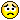1
|
8614 Views
|
3 Replies
|
2 Total Likes
View groups...
Share
GROUPS:

# Surface parametrization interpolation

Posted 11 years ago
 Hello,I am interested in studying the behavior of certain types of parametrizations on the sphere. However, I only know how to generate them discretely. I am able to refine the mesh to a higher and higher density, but I would like to actually interpolate the data that I have.My data are stored as a k  by n by 3 array of values. Each row corresponds to one of the parameter lines, and each column corresponds to the other parameter line. How do I provide a domain and interpolate this array into a surface parametrization?The key problems here are:1) I want my interpolation to pass through my data points *EXACTLY*.2) I want to specify the domain of my parameter variables.My initial thoughts:a) If I didn't care about the interpolation passing through the points, I could use a BSplineFunction[], but I want to gaurantee that my sample points are in the interpolation.b) I can't figure out how to get Interpolation[]  to take in data which are k by n by 3 and generate a parametrization. It seems like I need to have things in terms of Monge patches (i.e. {x, y, f(x,y)} but I can't write my data in that format).For example, given a sample of spherical coordinates, can we reconstruct a spherical coordinate parametrization of the sphere?Here is some code to get started:r[u_, v_] := N@{Cos[u] Sin[v], Sin[u] Sin[v], Cos[v]};spherePts = Table[r[u, v], {u, 0, 2 Pi, Pi/10}, {v, 0, Pi, Pi/20}];Show[Graphics3D@Sphere[], ListPointPlot3D[spherePts, PlotStyle -> {PointSize[0.05]}]](* How do I specify a different domain here? *)bsplineFunc = BSplineFunction[spherePts]ParametricPlot3D[bsplineFunc[u, v], {u, 0, 1}, {v, 0, 1}](* These two values should be equal to each other, if the bsplineFunc \passed through all the interpolation points exactly. *)bsplineFunc[1/2, 1/2] == spherePts[[11, 11]]bsplineFunc[1/2, 1/2]spherePts[[11, 11]]Any thoughts would be greatly appreciated. Best wishes,AndyBy the way: I apologize for the poor formatting of the post. I was unable to copy and paste my Mathematica code into the Mathematica textboxes. Instead, I had to first paste as text and then convert the text into Mathematica code. How do I insert the output of Mathematica code + images, for example?
3 Replies
Sort By:
Posted 11 years ago
 Andy, you actually formatted your post very well. We will take a look at the pasting procedure you described. In a meantime, if you have any suggestions about the site we would love to hear them through:Feedback button on the right of community pagesFeedback posted in the Wolfram Community groupThank you!
Posted 11 years ago
 BSplineFunction does not interpolate given points (control points), so of course the result is expected.You can create a list of grid points like this:spherePtsList = Flatten[Table[{i, j, spherePts[[i, j]]}, {i, 21}, {j, 21}], 1];Now, create an interpolation function based on that:f = Interpolation[spherePtsList]Now, test:In:= Table[f[i, i] === spherePts[[i, i]], {i, 1, 21}]Out= {True, True, True, True, True, True, True, True, True, True, True, True, True, True, True, True, True, True, True, True, True}And graphics:ParametricPlot3D[f[u, v], {u, 1, 21}, {v, 1, 21}]You can reparametrize it to be between 0 and 1.spherePtsList2 = Flatten[Table[N@{(i-1)/20,(j-1)/20,spherePts[[i,j]]},{i,21},{j,21}],1];g=Interpolation[spherePtsList2]Also, if you want to use B-spline, you can choose Method->"Spline" for the interpolation, but it may introduce a slight numerical error at the inpolation point (it will be equal (==) within machine precision, but not exactly the same (===)).Besides, the same problem here... Can't copy-and-paste inside the M- box....Posted 11 years ago
 Thank you Yu-Sung Chang for your thorough response. This was very helpful! Your initial response using ListInterpolation was also useful, because that strategy allows you to specify the domain in the ListInterpolation function call itself. Of course as you show I can do this by scaling the grid very easily before calling Interpolation :-)I have two follow-up questions:1) Is it possible to choose my own interpolation scheme for the ListInterpolation or Interpolation? (e.g. how NDSolve allows you to write your own function at each time step). As I would like to extend this Interpolation to use Spherical Linear Interpolation because I know that I am working constrained to the sphere (not necessarily unit). Here is a simple helper function which interpolates from point p0 to point p1. It would be interesting if Interpolation allowed for user-defined schemes between points.slerp[p0_, p1_] := Module[{angle},angle = ArcCos[Dot[p0, p1]/Norm[p0]/Norm[p1]];  Sin[(1 - t) angle]/Sin[angle] p0 + Sin[t angle]/Sin[angle] p1]2) Is it possible to recover a parametrization for a specific contour line / ContourPlot? (or the Boundary of a RegionPlot?) For example, if I now take my spherical parametrization and want to solve for (using your notation from above)ContourPlot[Last[g[u,v]]==0, {u, 0, 1}, {v, 0, 1}]OrRegionPlot[Last[g[u,v]] >= 0, {u, 0, 1}, {v, 0, 1}]Best wishes,Andy